# ISEE Lower Level Math : Geometry

## Example Questions

### Example Question #71 : Geometry

Find the area of a rectanlge whose length isand width is.Explanation:

To solve, simply use the formula for the area of a rectangle. Thus,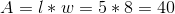### Example Question #72 : Geometry

A garden is 7 by 5 in size and you need to know how much fence is needed to surround it.  What is the perimeter?Explanation:

The perimeter is all four sides added together so you have two sides that areand two that are.  So the answer would be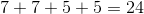.

### Example Question #73 : Geometry

A computer screen has a height of 9 inches and a width of 16 inches. Find its area.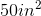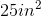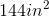Explanation:

A computer screen has a height of 9 inches and a width of 16 inches. Find its area.

Area can be found by multiplying the length by the width.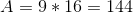So our answer is 144

### Example Question #41 : How To Find The Area Of A Rectangle

A rectangle measuresinches on its short side, and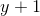inches on its long side.  What is its area?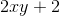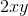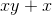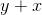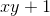Explanation:

To find the area of a rectangle, multiply the length of the long side by the length of the short side.  The best way to find the answer is: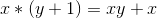### Example Question #42 : How To Find The Area Of A Rectangle

A rectangle measuresinches on its short side, and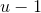inches on its long side.  What is its area?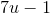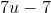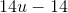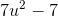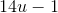Explanation:

To find the area of a rectangle, multiply the length of the long side by the length of the short side.  The best way to find the answer is: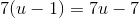### Example Question #43 : How To Find The Area Of A Rectangle

A swimming pool is in the shape of a rectangle that is 20 feet wide and 30 feet long.  Find the area of the swimming pool.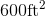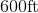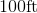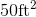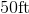Explanation:

To find the area of a rectangle, we use the following formula: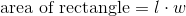where l is the length and is the width of the rectangle.

In this case, we know the length of the swimming pool is 30 feet.  We also know the width of the pool is 20 feet.  So, we can substitute into the formula.  We get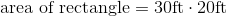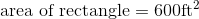### Example Question #44 : How To Find The Area Of A Rectangle

Use the following to answer the question.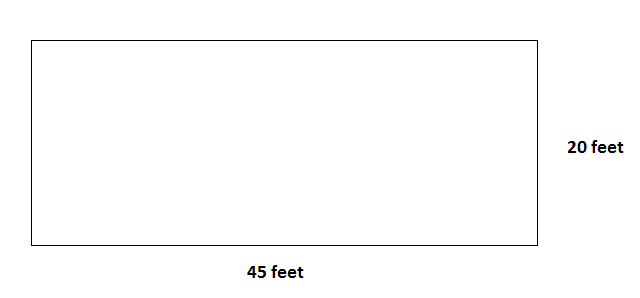You go to the community center to go swimming.  You see that the pool is a rectangular shape.  If the pool has a length of 45 feet and a width of 20 feet, what is the area of the pool?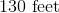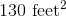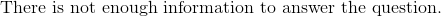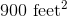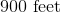Explanation:

To find the area of a rectangle, we use the following formula:where l is the length and w is the width of the rectangle.

So, given the rectanglewe can see the length is 45 feet and the width is 20 feet.  Knowing this, we can substitute into the formula.  We get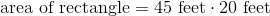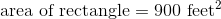### Example Question #45 : How To Find The Area Of A Rectangle

Find the area of a rectangle with a length of 10cm and a width that is half the length.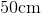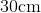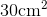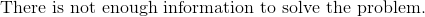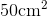Explanation:

To find the area of a rectangle, we will use the following formula:where l is the length and w is the width of the rectangle.

Now, we know the length of the rectangle is 10cm.  We also know the width is half of the length.  Therefore, the width is 5cm.  Knowing this, we can substitute into the formula.  We get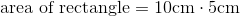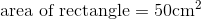### Example Question #46 : How To Find The Area Of A Rectangle

Find the area of a rectangle with a width of 6 feet and a length that is two times the width.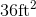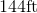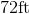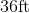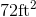Explanation:

To find the area of a rectangle, we will use the following formula:where l is the length and w is the width of the rectangle.

Now, we know the width is 6 feet.  We know the length is two times the width.  Therefore, the length is 12 feet.  Knowing this, we can substitute into the formula.  We get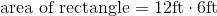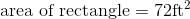### Example Question #47 : How To Find The Area Of A Rectangle

Find the area of a rectangle with a width of 4 inches and a length that is twice the width.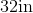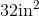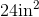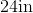Explanation:

To find the area of a rectangle, we will use the following formula:where l is the length and w is the width of the rectangle.

Now, we know the width of the rectangle is 4 inches.  We also know the length is two times the width.  Therefore, the length of the rectangle is 8 inches.  Knowing this, we can substitute into the formula.  We get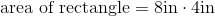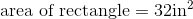### All ISEE Lower Level Math Resources